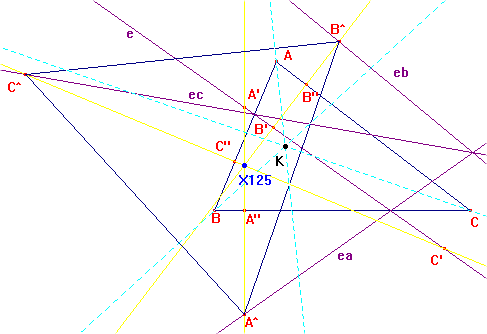r1560

Let ea, eb, ec be the reflections of the Euler lines in the sides. Let A', B', C' the orthogonal projections of the vertices on the Euler line; A", B", C" the orthogonal projections of A', B', C' on the sides; A^ the intersection between ea and A'A", B^, C^ its analogs. The triangles ABC and A^B^C^ are orthologic, with orthologic centers the symmedian K and the point X(125).